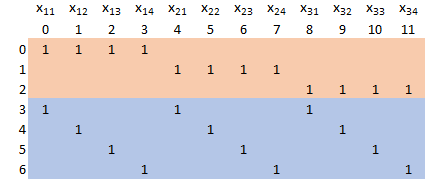## Monday, October 7, 2019

### Scipy linear programming: a large but easy LP

Scipy.optimize.linprog  recently added a sparse interior point solver . In theory we should be able to solve some larger problems with this solver. However the input format is matrix based. This makes it difficult to express LP models without much tedious programming. Of course if the LP model is very structured things are a bit easier. In  the question came up if we can solve some reasonable sized transportation problems with this solver. I claimed this new interior point solver should be able to tackle reasonably large transportation problems. As transportation problems translate into large but easy LPs (very sparse, network structure) this is a good example to try out this solver. It should not require too much programming.

An LP model for the transportation problem can look like:

Transportation Model
\begin{align} \min \> & \sum_{i,j} \color{darkblue}c_{i,j} \color{darkred} x_{i,j} \\ & \sum_j \color{darkred} x_{i,j} \le \color{darkblue}s_i &&\forall i\\ & \sum_i \color{darkred} x_{i,j} \ge \color{darkblue}d_j &&\forall j\\ & \color{darkred}x_{i,j}\ge 0\end{align}

Here $$i$$ indicate the supply nodes and $$j$$ the demand nodes. The problem is feasible if total demand does not exceed total supply (i.e. $$\sum_i s_i \ge \sum_j d_j$$).

Even if the transportation problem is dense (that is each supply node can serve all demand nodes or in other words each link $$i \rightarrow j$$ exists), the LP matrix is sparse. There are 2 nonzeros per column.LP Matrix

The documentation mentions we can pass on the LP matrix as a sparse matrix. Here are some estimates of the difference in memory usage:

100x100500x5001000x1000
Source Nodes1005001,000
Destination Nodes1005001,000
LP Variables10,000250,0001,000,000
LP Constraints2005002,000
LP Nonzero Elements20,000500,0002,000,000
Dense Memory Usage (MB)151,90715,258
Sparse Memory Usage (MB)0.37.630.5

For the $$1000\times 1000$$ case we see that a sparse storage scheme will be about 500 times as efficient.

Sparsity is very important both inside the solver as at the modeling level. Larger but sparser models are often to be preferred above smaller but denser models. Exploiting sparsity will not only save memory but also increase performance: by skipping zero elements we prevent doing all kind of useless work (like multiplying by zero or adding zeros).

#### Solving a 1000x1000 transportation problem: Implementation

• The package scipy.sparse  is used to form a sparse matrix. We use three parallel arrays to populate the sparse matrix: one integer array with the row numbers, one integer array with the column numbers and one floating point array with the values. All these arrays have the same length: the number of nonzeros in the LP matrix.
• Scipy.optimize.linprog does not allow for $$\ge$$ constraints. So our model becomes: \begin{align} \min &\sum_{i,j} c_{i,j} x_{i,j}\\ & \sum_j x_{i,j} \le s_i &&\forall i \\ & \sum_i -x_{i,j} \le -d_j &&\forall j\\ & x_{i,j}\ge 0\end{align}

When I run this, I see:

Primal Feasibility  Dual Feasibility    Duality Gap         Step             Path Parameter      Objective
1.0                 1.0                 1.0                 -                1.0                 4999334.387281
0.01096610265509    0.01096610265504    0.01096610265504    1.0              0.01096610265523    3423127.924532
0.007470719084731   0.007470719084695   0.007470719084695   0.3369198212982  0.007470719084826   1045138.710249
0.007375696439705   0.007375696439669   0.007375696439669   0.01405171378191 0.007375696439798   946062.4541516
0.006900523710037   0.006900523710004   0.006900523710004   0.07151611989327 0.006900523710125   631457.8940984
0.003392688227185   0.003392688227169   0.003392688227169   0.5542765654086  0.003392688227229   106030.5627759
0.002716216726218   0.002716216726205   0.002716216726205   0.2210823772546  0.002716216726252   77660.93708537
0.00151605426328    0.001516054263272   0.001516054263272   0.4706161702772  0.001516054263299   39012.6976106
0.001238382883199   0.001238382883193   0.001238382883193   0.2007381529847  0.001238382883215   31262.77924434
0.0006888763719364  0.000688876371933   0.0006888763719331  0.4711955496918  0.0006888763719452  16884.5788155
0.0004045311601541  0.0004045311601521  0.0004045311601522  0.4504577243574  0.0004045311601593  9812.570668161
0.0003278435563858  0.0003278435563842  0.0003278435563842  0.2062071599936  0.00032784355639    7943.50442653
0.0001938174872602  0.0001938174872593  0.0001938174872593  0.4304958950603  0.0001938174872627  4718.01892459
0.0001272127336263  0.0001272127336257  0.0001272127336257  0.371775562858   0.000127212733628   3126.320160308
7.325610966318e-05  7.325610966282e-05  7.325610966283e-05  0.4526986333113  7.325610966411e-05  1837.061691682
6.047737643405e-05  6.047737643373e-05  6.047737643375e-05  0.1896942778068  6.047737643482e-05  1530.292617672
3.301112106729e-05  3.301112106712e-05  3.301112106713e-05  0.4758440911431  3.301112106771e-05  870.6399411648
2.231615463384e-05  2.231615463375e-05  2.231615463374e-05  0.3562669388094  2.231615463413e-05  613.0954966036
1.300693055479e-05  1.300693055474e-05  1.300693055474e-05  0.4437694284722  1.300693055496e-05  388.3160007487
7.533045251385e-06  7.533045251368e-06  7.533045251357e-06  0.4485635094836  7.533045251489e-06  255.9636413848
3.799832196644e-06  3.799832196622e-06  3.799832196633e-06  0.5264643380152  3.7998321967e-06    165.5742065953
2.01284588862e-06   2.012845888624e-06  2.012845888615e-06  0.5006416028336  2.01284588865e-06   122.2520897954
1.143491145379e-06  1.143491145387e-06  1.143491145377e-06  0.4712206751612  1.143491145397e-06  101.1678772704
5.277850584407e-07  5.277850584393e-07  5.277850584402e-07  0.5711139027487  5.277850584494e-07  86.20125171613
3.125695105059e-07  3.125695105195e-07  3.125695105058e-07  0.4315945026363  3.125695105113e-07  80.96090171621
1.118500099738e-07  1.118500099884e-07  1.118500099743e-07  0.6743118743189  1.118500099763e-07  76.06812425522
4.412565084911e-08  4.412565086951e-08  4.412565085053e-08  0.6297257004579  4.412565085131e-08  74.41374033755
6.833044779903e-09  6.833044770544e-09  6.833044776856e-09  0.8682333453577  6.833044776965e-09  73.50145019804
3.3755500974e-10    3.375549807043e-10  3.375549865145e-10  0.9528386773998  3.375549866004e-10  73.34206256371
1.066148223577e-13  1.065916625724e-13  1.066069704785e-13  0.9998776987355  1.066069928771e-13  73.3337765897
7.763476236577e-18  3.543282811637e-17  5.469419174887e-18  0.9999500035089  5.330350298476e-18  73.3337739491
Optimization terminated successfully.
Current function value: 73.333774
Iterations: 30
Filename: transport.py

Line #    Mem usage    Increment   Line Contents
================================================
59     70.6 MiB     70.6 MiB   @profile
60                             def run():
61                                # dimensions
62     70.6 MiB      0.0 MiB      M = 1000  # sources
63     70.6 MiB      0.0 MiB      N = 1000 # destinations
64     78.3 MiB      7.7 MiB      data = GenerateData(M,N)
65    108.9 MiB     30.5 MiB      lpdata = FormLPData(data)
66    122.6 MiB     13.7 MiB      res = opt.linprog(c=np.reshape(data['c'],M*N),A_ub=lpdata['A'],b_ub=lpdata['rhs'],options={'sparse':True, 'disp':True})


This proves we can actually solve a $$1000 \times 1000$$ transportation problem (leading to an LP with a million variables) using standard Python tools.

#### Notes

It is noted that a dense transportation problem (with all links $$i \rightarrow j$$ allowed) produces a sparse LP. It is also possible the transportation problem is sparse: only some links are allowed. Sparse transportation problems are a little bit more difficult to set up: the LP matrix is less structured, so we need more advanced data structures (probably a dict to establish a mapping from each existing link  $$i \rightarrow j$$ to a column number). A good modeling tool may help here.

#### References

1.Thanks for post! Is it possible to solve 1000 x 1000 transportation problem in normal laptop? Could you please clarify this

1.The results from the memory profiier is shown, so you see can see *exactly* how much memory I needed for this run.

2.Good post! I'm having trouble with my numpy arrays trying to solve a LP problem with scipy and I will try out to formulate it with sparse matrices.

One question on how your output is shown. My scipy linprog output looks completely different and I can't see any informations about memory usage.
How did you activate those informations?

1.I used "from memory_profiler import profile" to allow for memory profiling.

2.Voce poderia me auxiliar a pegar as informaçoes da matriz

3.Is it possible to have access to the code? What does FormLPData(data) do?